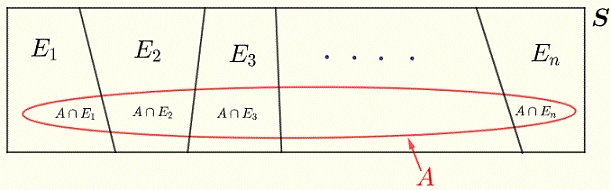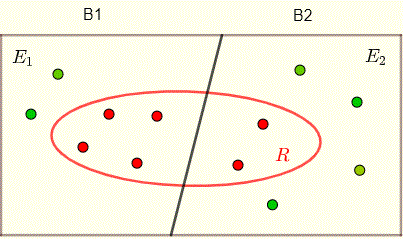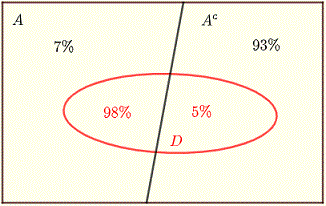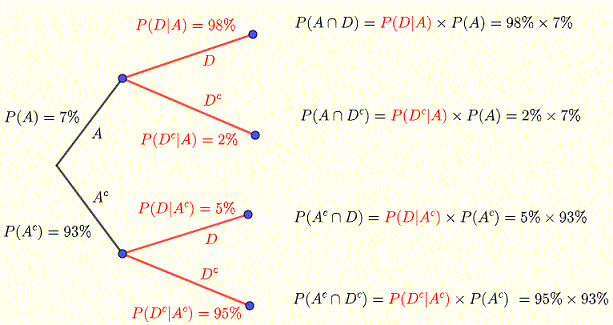# Bayer's Theorem Examples with Solutions

   

Bayes' theorem to find conditional porbabilities is explained and used to solve examples including detailed explanations. Diagrams are used to give a visual explanation to the theorem. Also the numerical results obtained are discussed in order to understand the possible applications of the theorem.

## Bayes' theorem

From law of total probability$P(A) = \sum_{i=1}^{n} P(A | E_i) P(E_i)$

The definition of
conditional probability is used to write

  $P(A) P(E_i | A) = P(E_i) P(A | E_i)$
which gives  
$P(E_i | A) = \dfrac{P(E_i) P(A | E_i)}{P(A)}$

Substitute $P(A)$ by the above sum to write Bayes' theorem as follows

$P(E_i | A) = \dfrac{P(E_i) P(A | E_i)}{\sum_{i=1}^{n} P(A | E_i) P(E_i)}$

## Use of Bayes' Thereom Examples with Detailed Solutions

Example 1 below is designed to explain the use of Bayes' theorem and also to interpret the results given by the theorem.
Example 1
One of two boxes contains 4 red balls and 2 green balls and the second box contains 4 green and two red balls. By design, the probabilities of selecting box 1 or box 2 at random are 1/3 for box 1 and 2/3 for box 2.
A box is selected at random and a ball is selected at random from it.
a) Given that the ball selected is red, what is the probability it was selected from the first box?
b) Given that the ball selected is red, what is the probability it was selected from the second box?
c) Compare the results in parts a) and b) and explain the answer.

Solution to Example 1
Let us call the first box B1 and the second box B2
Let event E1 be "select box 1" and event E2 "select box 2".
Let event R be "select a red ball".
All the above information is included in the diagram below.The probabilities of selecting one of the two boxes would are given (above) by
$P(E_1) = 1/3$ and $P(E_2) = 2/3$
The conditional probability that a selected ball is red given that it is selected from box 1 is given by
$P(R | E_1) = 4/6 = 2/3$ , 4 balls out of 6 are red in box 1
The conditional probability that a selected ball is red given that it is selected from box 2 is given by
$P(R | E_2) = 2/6 = 1/3$ , 2 balls out of 6 are red in box 2
a)
The question is to find the conditional probability that the ball is selected from box 1 given that it is red, is given by Bayes' theorem.
$P(E_1|R) = \dfrac{P(R | E1) P(E1) }{ P(R | E1) P(E1) + P(R | E2) P(E2) }$

$= \dfrac{ 2/3 * 1/3}{2/3 * 1/3 + 1/3 * 2/3} = 1/2$
b)
The question is to find the conditional probability that the ball is selected from box 2 given that it is red, is given by Bayes' theorem.
$P(E_2|R) = \dfrac{P(R | E2) P(E2) }{ P(R | E1) P(E1) + P(R | E2) P(E2) }$

$= \dfrac{ 1/3 * 2/3}{2/3 * 1/3 + 1/3 * 2/3} = 1/2$
c)
The two probabilities calculated in parts a) and b) are equal.
Although there are more red balls in box 1 than in box 2 (twice as much), the probabilities calculated above are equal because the probabilities of selecting box 2 is higher (twice as much) than the probability of selecting box 1. Bayes' theorem takes all the information into consideration.

Example 2
1% of a population have a certain disease and the remaining 99% are free from this disease. A test is used to detect this disease. This test is positive in 95% of the people with the disease and is also (falsely) positive in 2% of the people free from the disease.
If a person, selected at random from this population, has tested positive, what is the probability that she/he has the disease?

Solution to Example 2
Let D be the event "have the disease" and FD be the event "free from the disease"
Let the event TP be the event that the "test is positive".
A diagram with all the above information is shown below.The probability that a person has the disease given that it has tested positive is given by Bayes' theorem:
$P(D | TP) = \dfrac{P(TP | D) P(D) }{ P(TP | D) P(D) + P(TP | ND) P(ND) }$

$\quad \quad \quad = \dfrac{95\% 1\%}{95\% 1\% + 2\% 99\% } = 0.32$

Although a person tests positive, the probability of having the disease is quite low.
Explanation
Note that even when a person tests positive that does not mean that she/he has the disease; and that is because the number of disease free (99%) is much higher that those who have the disease (1%).
Let us clarify the results obtained above using some concrete numbers.
Suppose that 1000 people are tested
disease Free are: $99\% \times 1000 = 990$ and    $2\% \times 990 = 19.8 \approx 20$ test positive
People with disease: $1\% \times 1000 = 10$ and    $95\% \times 10 = 9.5 = 9.5 \approx 10$ test positive
Of all those who tested positive which are    20 + 10 = 30    only 10 have the disease.
Percentage of those who test positive but do not have the disease is give by: 9.5 / (19.8 + 9.5) = 0.32
which is the probability $P(D | TP)$ computed above.

Example 3
Three factories produce light bulbs to supply the market. Factory A produces 20%, 50% of the tools are produced in factories B and 30% in factory C.
2% of the bulbs produced in factory A, 1% of the bulbs produced in factory B and 3% of the bulbs produced in factory C are defective.
A bulb is selected at random in the market and found to be defective. what is the probability that this bulb was produced by factory B?

Solution to Example 3
Let $P(A) = 20\%$, $P(B) = 50\%$ and $P(C) = 30\%$ represent the probabilities that a bulb selected at random is from factory A, B and C respectively.
Let $P(D)$ be the probability that a defective bulb is selected.
Let $P(D | A) = 2\%$, $P(D | B) = 1\%$ and $P(D | C) = 3\%$ represent the conditional probabilities that a bulb is defective given that it is selected from factory A, B and C respectively.
We now calculate that the conditional probability that the bulb was produced by factory B given that it is defective written as $P(B | D)$ and given by Bayes' theorem.

$P(B | D) = \dfrac{P(D | B) P(B) }{ P(D | A) P(A) + P(D | B) P(B) + P(D | C) P(C)}$

$= \dfrac{1\% \times 50\%}{ 2\% \times 20\% + 1\% \times 50\% + 3\% \times 30\%} = 0.2777$

Although factory B produces 50% of the bulbs, the probability that the selected (defective) bulb comes from this factory is low because the bulbs produced by this factory have low probability (1%) of being defective.

Example 4
A radar system is designed such that the probability of detecting the presence of an aircraft in its range is 98%. However if no aircraft is present in its range it still report (falsely) that an aircraft is present with a probability of 5%. At any time, the probability that an aircraft is present within the range of the radar is 7%.
a) What is the probability that no aircraft is present in the range of the radar given that an aircraft is detected?
b) What is the probability that an aircraft is present in the range of the radar given that an aircraft is detected?
c) What is the probability that an aircraft is present in the range of the radar given that no aircraft is detected?
d) What is the probability that no aircraft is present in the range of the radar given that no aircraft is detected?

Solution to Example 4
We shall use the complement notations.
Let $A$ be the event that an aircraft is present and $A^c$ the event complement of $A$ meaning no aircraft is present.
The probability of A occurring is given as
$P(A) = 7\%$
and
$P(A^c) = 100\% - 7\% = 93\%$
Let $D$ be the event that an aircraft is detected by the radar system and $D^c$ the complement of $D$ meaning no aircraft is detected.
The conditional probability of that an aircraft is detected given that it is present is given above and is written as
$P(D | A) = 98%$
$P(D | A^c) = 5%$
a)
Put all the above information in a Venn diagram as shown below.Use Bayes' theorem to write the probability that no aircraft is present in the range of the radar given that an aircraft is detected

$P(A^c | D) = \dfrac{ P(D | A^c) P(A^c) }{ P(D | A^c) P(A^c) + P(D | A) P(A)}$

$= \dfrac{5\% \times 93\%}{5\% \times 93\% + 98\% \times 7\%} \approx 0.4040$

b)
Use Bayes' theorem to write the probability that an aircraft is present in the range of the radar given that an aircraft is detected
$P(A | D) = \dfrac{ P(D | A) P(A) }{ P(D | A) P(A) + P(D | A^c) P(A^c)}$

$= \dfrac{98\% \times 7\%}{98\% \times 7\% + 5\% \times 93\%} \approx 0.5960$

c)
We first need to calculate the following probabilities
$P(D^c | A) = 100\% - 98\% = 2\%$
$P(D^c | A^c) = 100\% - 5\% = 95\%$
Put all the above information in a Venn diagram as shown below.Use Bayes' theorem to write the probability that an aircraft is present in the range of the radar given that an no aircraft is detected

$P(A | D^c) = \dfrac{ P(D^c | A) P(A) }{ P(D^c | A) P(A) + P(D^c | A^c) P(A^c)}$

$= \dfrac{2\% \times 7\%}{2\% \times 7\% + 95\% \times 93\%} \approx 0.0016$

d)
Use Bayes' theorem to write the probability that no aircraft is present in the range of the radar given that an no aircraft is detected

$P(A^c | D^c) = \dfrac{ P(D^c | A^c) P(A^c) }{ P(D^c | A^c) P(A^c) + P(D^c | A) P(A)}$

$= \dfrac{95\% \times 93\%}{95\% \times 93\% + 2\% \times 7\%} \approx 0.9984$

NOTE All the above calculations may be done with the help of a tree diagram shown below. Once the tree diagram have all the probabilities, it is easier to use these probabilities in Bayes' theorem in order to evaluate the final results.### More References and links

More on Probabilities .
conditional probability
law of total probability
Binomial Probabilities Examples and Questions
addition rule of probabilities
multiplication rule of probabilities
probability questions
classical formula for probability
mutually exclusive events